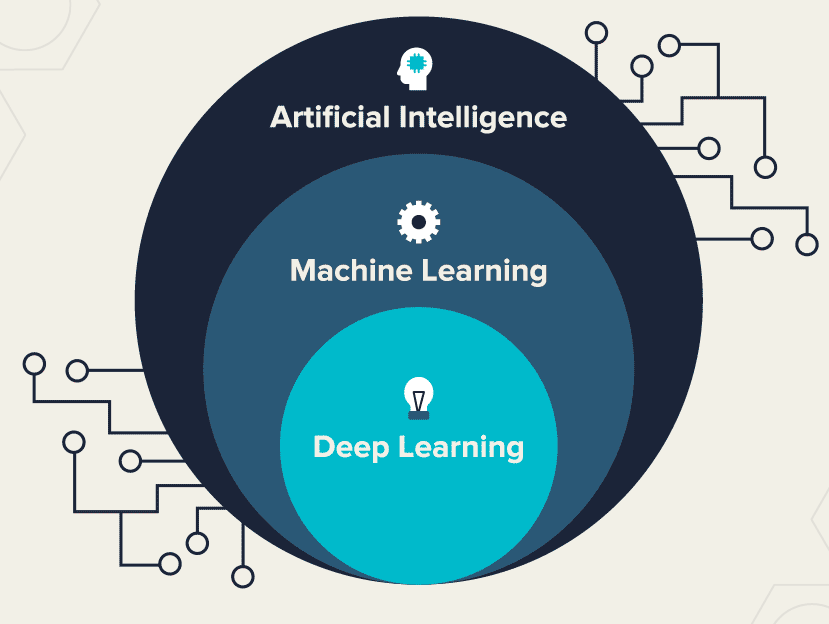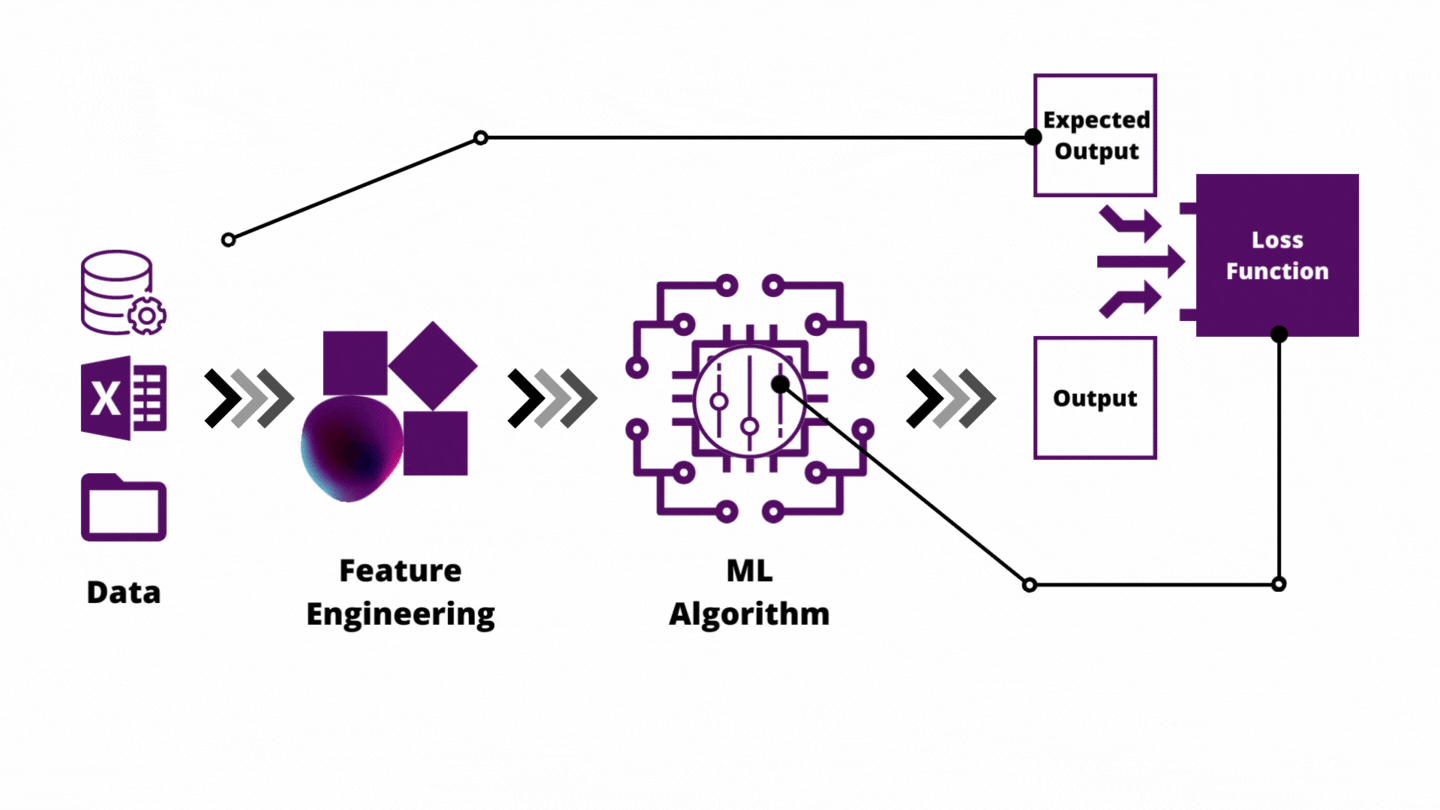# Machine Learning (ML)

Machine learning (ML) is a field devoted to understanding and building methods that let machines "learn" – that is, methods that leverage data to improve computer performance on some set of tasks. It is seen as a broad subfield of artificial intelligence. Machine learning algorithms build a model based on sample data, known as training data, in order to make predictions or decisions without being explicitly programmed to do so. - Machine learning | WikipediaLet’s take a moment to point out the main building blocks of a machine learning algorithm. The complete process of machine learning can be observed as a pipeline. At the beginning of that pipeline is, of course, data. Data can come from different sources. Other systems can generate it or humans can create it. Sometimes we use web scrappers to get the data from the web, while other times data is available in some database. More often than not, this data is unstructured and sparse. This can be a problem for algorithms, which is why the second step of the pipeline is pre-processing of the data and Feature Exploration/Learning. After data is preprocessed and prepared, we feed it to our machine learning algorithm. This is called the training process, during which the algorithm needs to learn. The machine learning algorithm itself contains different parameters within itself which make this learning possible. What do we mean by this? Well, based on these input values the algorithm creates predictions – output values. However, those predictions are not the only output that this algorithm provides.In Supervised Learning, we have the expected output, so we can use it to calculate how much predictions deviate from the expected results. For this, we can use different functions and measure the penalty in different ways. This function is called the loss function and the goal of the algorithm is to minimize the penalty calculated by this function. In mathematics, the expression we minimize or maximize is called an objective function. Then we use the calculated penalty to change the parameters of the machine learning algorithm to get better predictions next time. In general, this is done by minimizing the penalty we calculated. That is how the machine learning algorithm, not only produces the output values during the training process, but it changes itself so it can improve its predictions. That is how the implicit output of a machine learning algorithm is the algorithm itself. The trained algorithm is usually called the model. This name comes from mathematics. - [Introduction to Machine Learning with C# and ML.NET - Rubik's Code